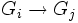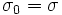# Chain-extensible automorphism

## Contents

BEWARE! This term is nonstandard and is being used locally within the wiki. [SHOW MORE]
This article defines an automorphism property, viz a property of group automorphisms. Hence, it also defines a function property (property of functions from a group to itself)
View other automorphism properties OR View other function properties
This term is related to: Extensible automorphisms problem
View other terms related to Extensible automorphisms problem | View facts related to Extensible automorphisms problem

## Definition

### Definition with symbols

An automorphism$\sigma$ of a group$G$ is termed chain-extensible if the following holds. Given the following data:

• A totally ordered set$I$ with unique minimum element$0$
• A group$G_i$ for each$i \in I$ such that$G_0 = G$
• Inclusions$G_i \to G_j$ for$i < j$, compatible with composition

There exist automorphisms$\sigma_i$ of$G_i$ for each$i$ such that whenever$i < j$ the restriction of$\sigma_j$ to$G_i$ is$\sigma_i$, and such that$\sigma_0 = \sigma$.